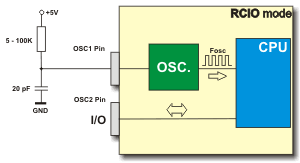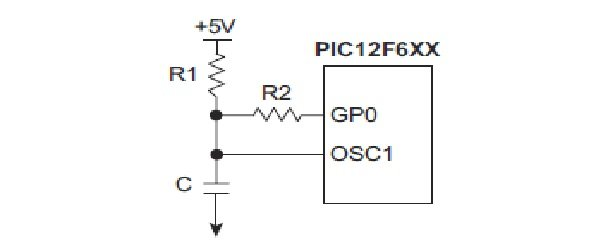# Dual speed RC oscillator for pic microcontroller

In this tutorial, I will show you how to design dual speed RC oscillator for pic micrcontroller. If you are designing a pic microcontroller based project where high frequency is not a requirement, there is no need to use external crystal oscillator. you can use internal oscillator of microcontroller. you can check this article to know how to select internal oscillator of pic microcontroller. if your required microcontroller do not have internal oscillator, you can use external oscillator as dual speed oscillator.

What does I mean by dual speed RC oscillator? Its mean you can either use RC oscillator as a lower speed or high speed oscillator just by changing the current flow through dual speed RC oscillator. In this tutorial, I will show you how to do it. To design a RC oscillator we need one resistor and one capacitor. But to design a dual speed RC oscillator, we need two resistors and one capacitor. One resistor will be used to change the frequency from low to high and high to low by changing the current flow through the other resistor as shown below. Usually we use only one resistor and one capacitor to design RC oscillator for pic microcontroller as shown below:Circuit diagram of dual speed RC oscillator is shown below.In dual speed RC oscillator, we have used to two resistors. To use RC oscillator as a dual speed oscillator , you need use one GPIO ( general purpose input and output pin of pic microcontroller) . Follow these steps to configure this RC oscillator as dual frequency mode:

• First of all use one input and output pin of microcontroller with resistor R2 as shown above. this pin will be used both as a input and output through programming.
• First when turn on microcontroller, this GPIO pin will be in high impedance state.
• Now declare this in and output pin using this command TRIS.GP0=0;  and make this pin high using this command PORT.GP0=1;
• Frequency of oscillator depends on values of R1, R2 and C capacitor.
• you can make calculations to get desire frequency. Many online calculators are available for this purpose.
• Frequency of pic microcontroller with external RC oscillator depends on values of resistance and capacitance on OSC1 pin.
• we change the frequency by changing the resistance value.
• Resistance value can be changed by changing voltage value on GP0 pin.
• When GP0 pin is high, resistor R1 becomes parallel to resistor R2 and it reduces the resistance.
• Decrease in resistance, increases the frequency of RC oscillator.
• When we use GP0 pin as a input by using this command TRIS.GP0=1 and it minimizes the current flows through resistor R2 .
• Now resistor R2 is no more in parallel with resistor R1 and resistance increases.
• The increase in resistance, decreases the frequency of RC oscillator.

So this how we can use dual speed RC oscillator with pic microcontroller. when frequency is low, power consumption will also be low. so when your requirement is high speed processing you can use GP0 pin as a output high and when your requirement is low power consumption, you can use GP0 pin as a input to operate microcontroller at low frequency.